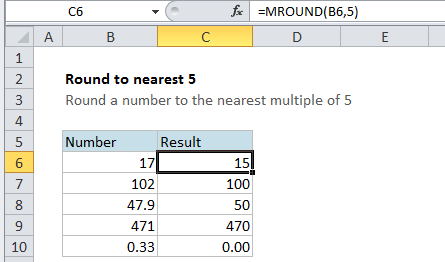## Excel Office

Excel How Tos, Tutorials, Tips & Tricks, Shortcuts

# Round to nearest 5 in Excel

This tutorials shows how to Round to nearest 5 in Excel.

If you need to round a number to the nearest multiple of 5, you can use the MROUND function and supply 5 for number of digits.

Worked Example:   Round a number up to nearest multiple in Excel

## Formula

`=MROUND(number,5)`## Explanation

In the example, cell C6 contains this formula:

`=MROUND(B6,5)`

The value in B6 is 17 and the result is 15 since 15 is the nearest multiple of 5 to 17.

Worked Example:   Round a number down to nearest multiple in Excel

### Other multiples

As you’d expect, you can use MROUND to round to other multiples as well:

```=MROUND(number,10) // nearest multiple of 10
=MROUND(number,50) // nearest multiple of 50
=MROUND(number,.05) // nearest 5 cents```

And so on.

Worked Example:   Excel MROUND, CEILING and FLOOR function examples

### Force up or down

You can use the CEILING function to force rounding up to the nearest multiple and the FLOOR function to force rounding down to the nearest multiple.